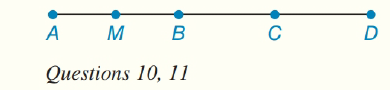Chapter 1.CT, Problem 10CT### Elementary Geometry for College St...

6th Edition
Daniel C. Alexander + 1 other
ISBN: 9781285195698

#### Solutions

Chapter
Section### Elementary Geometry for College St...

6th Edition
Daniel C. Alexander + 1 other
ISBN: 9781285195698
Textbook Problem
1 views

# In the figure A - B - C - D , and M is the midpoint of A B ¯ . If A B = 6.4   inches and B D = 7.2   inches , find M D ._________To determine

To find:

The value of MD.

Explanation

Given:

M is the midpoint of AB¯ and AB=6.4inches and BD=7.2inches

The given figure is,

Approach:

According to Segment-Addition Postulate, if two points A, B are given on a line segment, then third point C lies on the line segment if and only if,

AC+CB=AB.

Calculation:

Since, M is the midpoint of AB¯

### Still sussing out bartleby?

Check out a sample textbook solution.

See a sample solution

#### The Solution to Your Study Problems

Bartleby provides explanations to thousands of textbook problems written by our experts, many with advanced degrees!

Get Started

#### Find more solutions based on key concepts